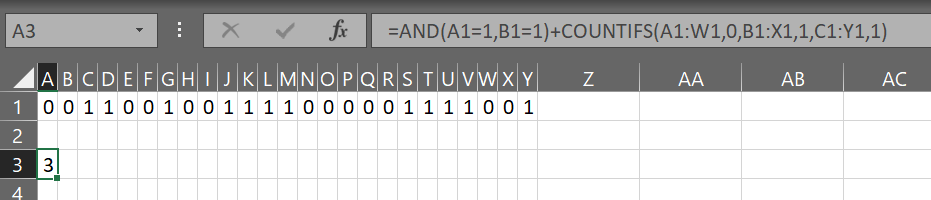# [Solved] Count number of times value appears consecutively in a row – Excel

Use:

``````=AND(A1=1,B1=1)+COUNTIFS(A1:W1,0,B1:X1,1,C1:Y1,1)
``````

We want to count where the there is a pattern of `0,1,1` hence the equal size but offset ranges. It will count where the pattern matches. We also want to count if `A1` and `B1` both equal `1` because it should be positive but does not meet the pattern.solved Count number of times value appears consecutively in a row – Excel